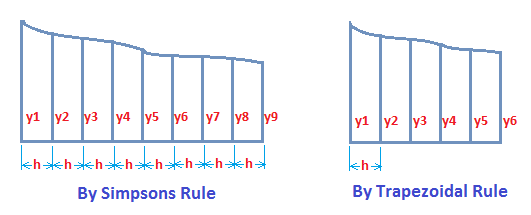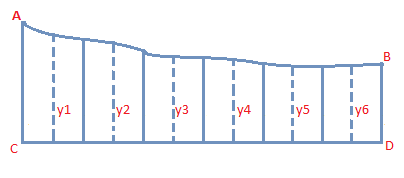# Area of Irregular Figures

There are various methods of calculating area of irregular figures. These methods give only approximate results. Some of the important methods are given below:

## By Simpson's Rule

Divide the base of figure into even number of equal parts and elongate ordinates on each part as shown in the below figure.

Let h = distance between each ordinate

Then, Area = $\frac{h}{3}$ [(y1 + y9) + 2(y3 +y5 + y7) + 4(y2 + y4 + y6 + y8)].## By Trapezoidal Rule

Divide the base of the figure in equal number of parts and draw ordinates from each of these points as shown below.

If y1, y2..., y6 are the lengths of ordinates and A = distance between each ordinate then

Area = h [$\frac{1}{2}$ (y1 + y6) + y2 + y3 + y4 + y5]

## Planimeter

With the help of an instrument known as planimeter, accurate areas can be found very quickly.

## Method of counting Squares

Draw the actual irregular diagram on a graph paper to some scale and count the number of squares covered by this diagram, taking those squares greater than one-half as one, and neglecting those having less than one-half of then as covered by the diagram. This gives approximate areas and is used where much accuracy is not desired.

Area of figure = No. of squares x Area of one square.

## Area of Trapezium

Area of trapezium = Distance between successive ordinates x Sum of half first and last ordinates, together with all the remaining ordinates.

## The Mid-ordinate Method

Divide the base line of the figure into any number of small equal parts and at the centre of each of these parts, draw ordinates as shown below. Calculate the average length of these ordinates and multiply by the length of base line.

This method also gives approximate area.Area = $\frac{(Y_1 + Y_2 + Y_3 + Y_4 + Y_5 + Y_6) \times CD }{6}$

Average of Length of mid ordinates x Length of base line.

Next Chapters

Latest Articles

Average Acceleration Calculator

Average acceleration is the object's change in speed for a specific given time period. ...

Free Fall Calculator

When an object falls into the ground due to planet's own gravitational force is known a...

Permutation

In Mathematics, the permutation can be explained as the arrangement of objects in a particular order. It is an ordered...

Perimeter of Rectangle

A rectangle can be explained as a 4-sided quadrilateral which contains equal opposite sides. In a rectangle

Perimeter of Triangle

A three sided polygon which has three vertices and three angles is called a triangle. Equilateral triangle...